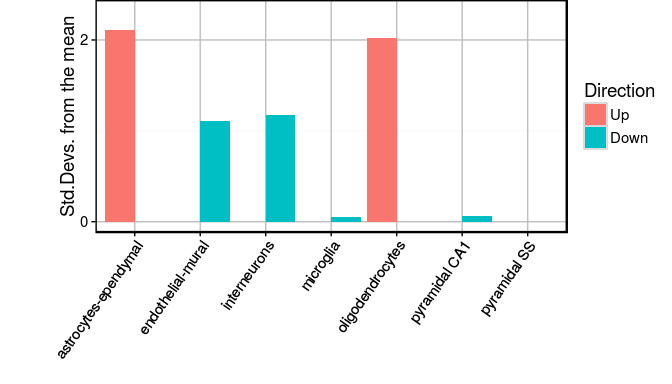# 1 Citation

If you use EWCE1 in published research, please cite N. Skene et al (2016).

N.G. SKENE, S.G.N. GRANT
"Expression weighted cell type enrichments reveal genetic and cellular nature of major brain disorders."
Frontiers in Neuroscience 2016

# 2 Introduction

The EWCE package is designed to facilitate expression weighted celltype enrichment analysis as described in our Frontiers in Neuroscience paper1.

The package was originally designed to work with the single cell cortical transcriptome data from the Linnarsson lab2 which is available at http://linnarssonlab.org/cortex/. Using this package it is possible to read in any single cell transcriptome data, provided that it is formatted in the same manner as this dataset.

The EWCE process involves testing for whether the genes in a target list have higher levels of expression in a given cell type than can reasonably be expected by chance. The probability distribution for this is estimated by randomly generating gene lists of equal length from a set of background genes.

The EWCE method can be applied to any gene list. In the paper we reported it’s application to genetic and transcriptomic datasets, and in this vignette we detail how this can be done.

Note that throughout this vignette we use the terms ‘cell type’ and ‘sub-cell type’ to refer to two levels of annotation of what a cell type is. This is described in further detail in our paper1, but relates to the two levels of annotation provided in the Linnarsson dataset2. In this dataset a cell is described as having a cell type (i.e. ‘Interneuron’) and subcell type (i.e. ‘Int11’ a.k.a Interneuron type 11).

# 3 Overview

The process for using EWCE essentially involves three steps.

First, one needs to load the relevant single cell transcriptome dataset. Single cell transcriptome data is read in from a text file using the read_celltype_data.

The user then obtains a gene set and a suitable background gene set. As the choice of gene sets is up to the user we do not provide functions for doing this. Appropriate choice of background set is discussed in the associated publication.

Bootstrapping is then performed using the bootstrap.enrichment.test function.

# 4 Installing EWCE

The EWCE package is available from the Bioconductor repository at http://www.bioconductor.org To be able to install the package one needs first to install R and the core Bioconductor packages. If you have already installed Bioconductor packages on your system then you can skip the two lines below.

source("http://bioconductor.org/biocLite.R")
biocLite()

Once the core Bioconductor packages are installed, we can install the EWCE package by

source("http://bioconductor.org/biocLite.R")
biocLite("EWCE")

You can then load the package:

library(EWCE)

The first step for all analyses is to load the single cell transcriptome (SCT) data. For the purposes of this example we will use the dataset described in “Cell types in the mouse cortex and hippocampus revealed by single-cell RNA-seq”, Science, 2015. The following code downloads this file and then passes it to the read_celltype_data function. This functions loads the data, calcuates the mean expression in each subcell type, and then for each gene assigns a relative proporotion of expression to each subcell and cell type.

download.file("http://linnarssonlab.org/blobs/cortex/expression_mRNA_17-Aug-2014.txt",
destfile="expression_mRNA_17-Aug-2014.txt")
path = "expression_mRNA_17-Aug-2014.txt"
celltype_data = read_celltype_data(path)

If you wish to load your own SCT dataset then just format it in a manner mirroring the dataset used above, and load it with the function. The same number of rows and columns proceeding the expression data will need to be used.

Because it is quite slow to run the read_celltype_data function we recommend saving the output.

save(celltype_data,file="celltype_data.rda")

While not required for further analyses it helps to understand what the outputs of this function are. Using the ggplot2 package to visualise the data, let us examine the expression of a few genes. If you have not already done so you will need to first install the ggplot2 package with install.packages("ggplot2"). For this example we use a subset of the Linnarsson cortex SCT dataset, which is accessed using data(celltype_Data). We recommend that you use the code above to regenerate this though and drop the data command from the below section.

set.seed(1234)
library(ggplot2)
library(reshape2)
data("celltype_data")
genes = c("Apoe","Gfap","Gapdh")
exp = melt(cbind(celltype_data$all_scts[genes,],genes),id.vars="genes") colnames(exp) = c("Gene","Cell","AvgExp") ggplot(exp)+geom_bar(aes(x=Cell,y=AvgExp),stat="identity")+facet_grid(Gene~.)+ theme(axis.text.x = element_text(angle = 90, hjust = 1))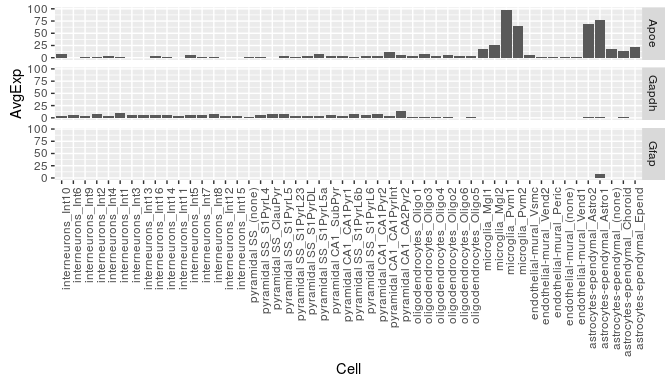This graph shows the average expression of three genes: Apoe, Gfap and Gapdh. While there are substantial differences in which cell types express these genes, the dominant effect seen here is the overall expression level of the data. For the purposes of this analysis though, we are not interested in overall expression level and only wish to know about the proportion of a genes expression which is found in a particular celltype. We can study this instead using the following code which examines the data frame celltype_data$subcell_dists:

exp = melt(cbind(celltype_data$subcell_dists[genes,],genes),id.vars="genes") colnames(exp) = c("Gene","Cell","ExpProp") ggplot(exp)+geom_bar(aes(x=Cell,y=ExpProp*100),stat="identity")+facet_grid(Gene~.)+ theme(axis.text.x = element_text(angle = 90, hjust = 1))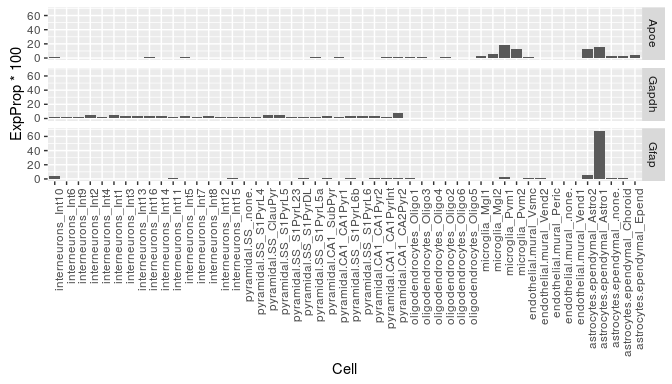We can now see in this graph that Gfap is the most specific to a cell type (Type 1 Astrocytes) of either of those three genes, with over 60% of it’s expression found in that cell type. It can also be seen that the majority of expression of Gapdh is in neurons but because their are a greater number of neuronal subtypes, the total expression proportion appears lower. We can examine expression across celltype level annotations by looking at celltype_data$cell_dists:

mouse.bg  = unique(setdiff(m2h$MGI.symbol,mouse.hits)) The target list is now converted to MGI symbols: print(mouse.hits) ##  "Sorl1" "Apoe" "Ms4a6c" "Bin1" "Cass4" "Ms4a6b" "Zcwpw1" ##  "Clu" "H2-Eb1" "Cd2ap" "Celf1" "Ms4a6d" "H2-Eb2" "Ptk2b" ##  "Nme8" "Ms4a4a" "Picalm" "Slc24a4" "Abca7" "Cd33" "Inpp5d" ##  "Siglech" "Fermt2" "Mef2c" And we have 18044 genes in background set. ## 6.2 Setting analysis parameters We now need to set the parameters for the analysis. For a publishable analysis we would want to generate over 10000 random lists and determine their expression levels, but for computational speed let us only use reps=1000. We then need to decide whether to test for enrichment in cell type or subcell type annotations: for this example we set subCellStatus=TRUE. reps=1000 # <- Use 1000 bootstrap lists so it runs quickly, for publishable analysis use >10000 subCellStatus=TRUE # <- Use subcell level annotations (i.e. Interneuron type 3) ## 6.3 Running EWCE analysis on genetic data We have now loaded the SCT data, prepared the gene lists and set the parameters. We run the model as follows: # Bootstrap significance testing, without controlling for transcript length and GC content full_results = bootstrap.enrichment.test(sct_data=celltype_data,mouse.hits=mouse.hits, mouse.bg=mouse.bg,reps=reps,sub=subCellStatus) The main table of results is stored in full_results$results. We can see the most significant results using:

print(full_results$results[order(full_results$results$p),3:5][1:6,]) ## p fold_change sd_from_mean ## microglia_Mgl2 0.000 81.03831 18.342022 ## microglia_Pvm1 0.000 208.92151 59.672642 ## astrocytes.ependymal_Astro2 0.000 88.05000 22.970246 ## microglia_Pvm2 0.001 133.14797 19.134990 ## microglia_Mgl1 0.002 40.70405 6.470147 ## astrocytes.ependymal_Astro1 0.002 58.25271 4.769903 The results can be visualised using another function, which shows for each cell type, the number of standard deviations from the mean the level of expression was found to be in the target gene list, relative to the bootstrapped mean: print(ewce.plot(full_results$results,mtc_method="BH"))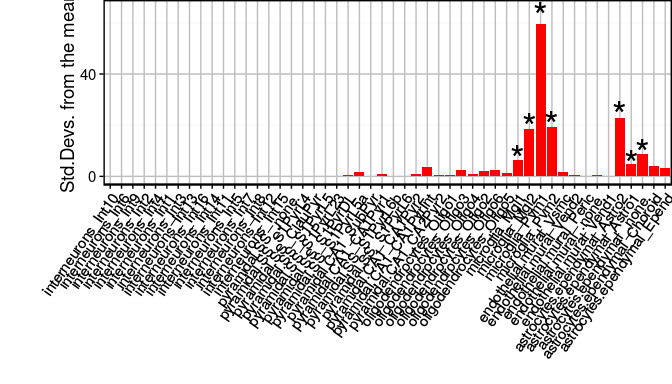If you want to view the characteristics of enrichment for each gene within the list then the generate.bootstrap.plots function should be used. This saves the plots into the BootstrapPlots folder. This takes the results of a bootstrapping analysis so as to only generate plots for significant enrichments. The listFileName argument is used to give the generated graphs a particular file name.

generate.bootstrap.plots(sct_data=celltype_data,mouse.hits=mouse.hits,mouse.bg=mouse.bg,reps=100,sub=subCellStatus,full_results=full_results,listFileName="VignetteGraphs")

## 6.4 Running EWCE analysis on genetic data with controls for transcript length and GC-content

When analysing genes found through genetic association studies it is important to consider biases which might be introduced as a result of transcript length and GC-content. The package can control for these by selecting the bootstrap lists such that the ith gene in the random list has properties similar to theith gene in the target list. To enable the algorithm to do this it needs to be passed the gene lists as HGNC symbols rather than MGI.

human.hits = unique(m2h[m2h$HGNC.symbol %in% example_genelist,"HGNC.symbol"]) human.bg = unique(setdiff(m2h$HGNC.symbol,human.hits))

The bootstrapping function then takes different arguments:

# Bootstrap significance testing controlling for transcript length and GC content
cont_results = bootstrap.enrichment.test(sct_data=celltype_data,human.hits=human.hits,
human.bg=human.bg,reps=reps,sub=subCellStatus,geneSizeControl=TRUE)

We plot these results using ewce.plot:

print(ewce.plot(cont_results$results,mtc_method="BH"))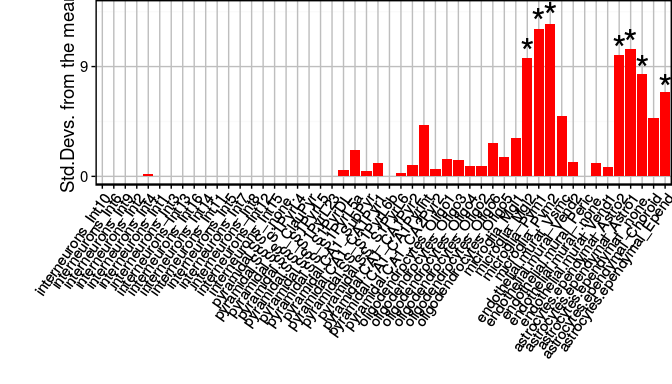This shows that the controlled method generates enrichments only marginally different results to the standard method. ## 6.5 Running EWCE analysis on cell type level annotations Both the analyses shown above were run on sub-cell type level analysis. It is possible to test on the smaller set of cell type level annotations by changing one of the arguments. # Bootstrap significance testing controlling for transcript length and GC content cont_results = bootstrap.enrichment.test(sct_data=celltype_data,human.hits=human.hits, human.bg=human.bg,reps=reps,sub=FALSE,geneSizeControl=TRUE) print(ewce.plot(cont_results$results,mtc_method="BH"))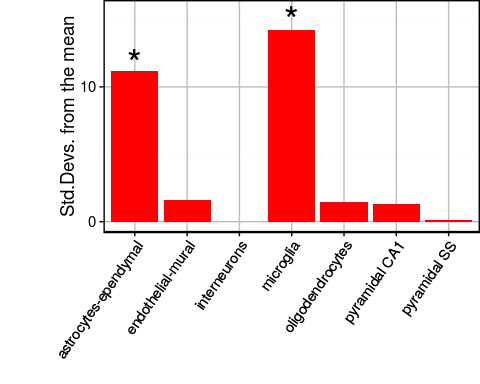With the subcell analysis each microglial subtype was enriched and correspondingly we see here that the microglial celltype is enriched.

## 6.6 Plotting results from multiple gene lists

It is often useful to plot results from multiple gene list analyses together. The ewce.plot function allows multiple enrichment analyses to be performed together. To achieve this the results data frames are just appended onto each other, with an additional list column added detailing which analysis they relate to.

To demonstrate this we need to first generate a second analysis so let us sample thirty random genes, and run the bootstrapping analysis on it.

gene.list.2 = mouse.bg[1:30]
second_results = bootstrap.enrichment.test(sct_data=celltype_data,mouse.hits=gene.list.2,
mouse.bg=mouse.bg,reps=reps,sub=subCellStatus)
full_res2 = data.frame(full_results$results,list="Alzheimers") scnd_res2 = data.frame(second_results$results,list="Second")
merged_results = rbind(full_res2,scnd_res2)
print(ewce.plot(total_res=merged_results,mtc_method="BH"))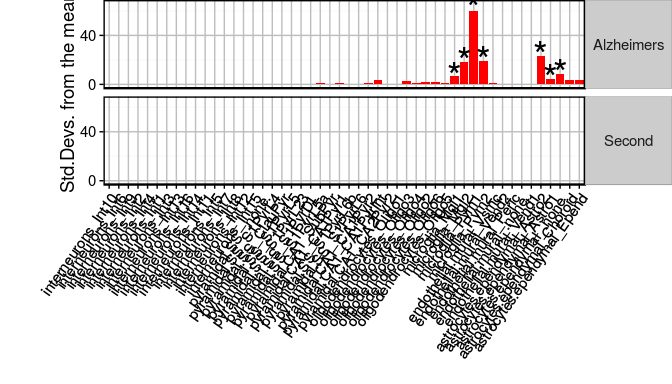As expected, the second randomly generated gene list shows no significant enrichments.

# 7 Application to transcriptomic data

## 7.1 Analysing single transcriptome study

For the prior analyses the gene lists were not associated with any numeric values or directionality. The methodology for extending this form of analysis to transcriptomic studies simply involves thresholding the most upregulated and downregulated genes.

To demonstrate this we have an example dataset tt_alzh. This data frame was generated using limma from a set of post-mortem tissue samples from brodmann area 46 which were described in a paper by the Haroutunian lab3.

The first step is to load the data, obtain the MGI ids, sort the rows by t-statistic and then select the most up/down-regulated genes. The package then has a function ewce_expression_data which thresholds and selects the gene sets, and calls the EWCE function. Below we show the function call using the default settings, but if desired different threshold values can be used, or alternative columns used to sort the table.

data(tt_alzh)
tt_results = ewce_expression_data(sct_data=celltype_data,tt=tt_alzh)

The results of this analysis can again be plotted using the ewce.plot function.

ewce.plot(tt_results\$joint_results)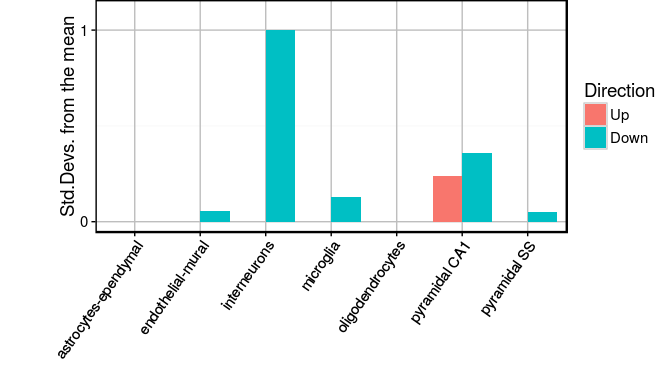As was reported in our paper, neuronal genes are found to be downregulated while glial genes are upregulated.

## 7.2 Merging multiple transcriptome studies

Where multiple transcriptomic studies have been performed with the same purpose, i.e. seeking differential expression in dlPFC of post-mortem schizophrenics, it is common to want to determine whether they exhibit any shared signal. EWCE can be used to merge the results of multiple studies.

To demonstrate this we use a two further Alzheimer’s transcriptome dataset coming from Brodmann areas 36 and 44: these area stored in tt_alzh_BA36 and tt_alzh_BA44. The first step is to run EWCE on each of these individually and store the output into one list.

# Load the data
data(tt_alzh_BA36)
data(tt_alzh_BA44)

# Run EWCE analysis
tt_results_36 = ewce_expression_data(sct_data=celltype_data,tt=tt_alzh_BA36)
tt_results_44 = ewce_expression_data(sct_data=celltype_data,tt=tt_alzh_BA44)

# Fill a list with the results
print(merged_res)
The results can then be plotted as normal using the ewce.plot function.
print(ewce.plot(merged_res))